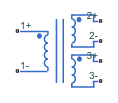# Three-Winding Mutual Inductor

Three coupled inductors

•Libraries:
Simscape / Electrical / Passive / Transformers

## Description

The Three-Winding Mutual Inductor block represents a set of three coupled inductors or windings. The voltage across the three windings is

`$\begin{array}{l}{V}_{1}={L}_{1}\frac{d{I}_{1}}{dt}+{M}_{12}\frac{d{I}_{2}}{dt}+{M}_{13}\frac{d{I}_{3}}{dt}\\ {V}_{2}={M}_{12}\frac{d{I}_{1}}{dt}+{L}_{2}\frac{d{I}_{2}}{dt}+{M}_{23}\frac{d{I}_{3}}{dt}\\ {V}_{3}={M}_{13}\frac{d{I}_{1}}{dt}+{M}_{23}\frac{d{I}_{2}}{dt}+{L}_{3}\frac{d{I}_{3}}{dt}\end{array}$`

where:

• Vi is voltage across the ith winding.

• Ii is current through the ith winding.

• Li is self inductance of the ith winding.

• Mij is mutual inductance of the ith and jth windings, ${M}_{ij}={K}_{ij}\sqrt{{L}_{i}{L}_{j}}$.

In the preceding equations, currents are positive when flowing into the positive node of their respective inductor terminals.

When you run a simulation that includes this block, the software checks the specified parameter values to ensure that the resulting device is passive. If it is not, the software issues an error.

## Ports

### Conserving

expand all

Electrical conserving port associated with the positive voltage of the first mutual inductor.

Electrical conserving port associated with the negative voltage of the first mutual inductor.

Electrical conserving port associated with the positive voltage of the second mutual inductor.

Electrical conserving port associated with the negative voltage of the second mutual inductor.

Electrical conserving port associated with the positive voltage of the third mutual inductor.

Electrical conserving port associated with the negative voltage of the third mutual inductor.

## Parameters

expand all

Self inductance of the first winding.

Self inductance of the second winding.

Self inductance of the third winding.

Coefficient that defines the mutual inductance between the first and second windings. The absolute value must be between 0 and 1, exclusive.

Coefficient that defines the mutual inductance between the first and third windings. The absolute value must be between 0 and 1, exclusive.

Coefficient that defines the mutual inductance between the second and third windings. The absolute value must be between 0 and 1, exclusive.

Select one of the following options for specifying an initial condition:

• `No` — Do not specify an initial condition for the model. This is the default option.

• `Yes` — Specify the initial inductor currents.

The current flowing through the first winding at the start of the simulation.

#### Dependencies

This parameter is visible only when you select `Yes` for the Specify initial condition parameter.

The current flowing through the second winding at the start of the simulation.

#### Dependencies

This parameter is visible only when you select `Yes` for the Specify initial condition parameter.

The current flowing through the third winding at the start of the simulation.

#### Dependencies

This parameter is visible only when you select `Yes` for the Specify initial condition parameter.

## Version History

Introduced in R2008a